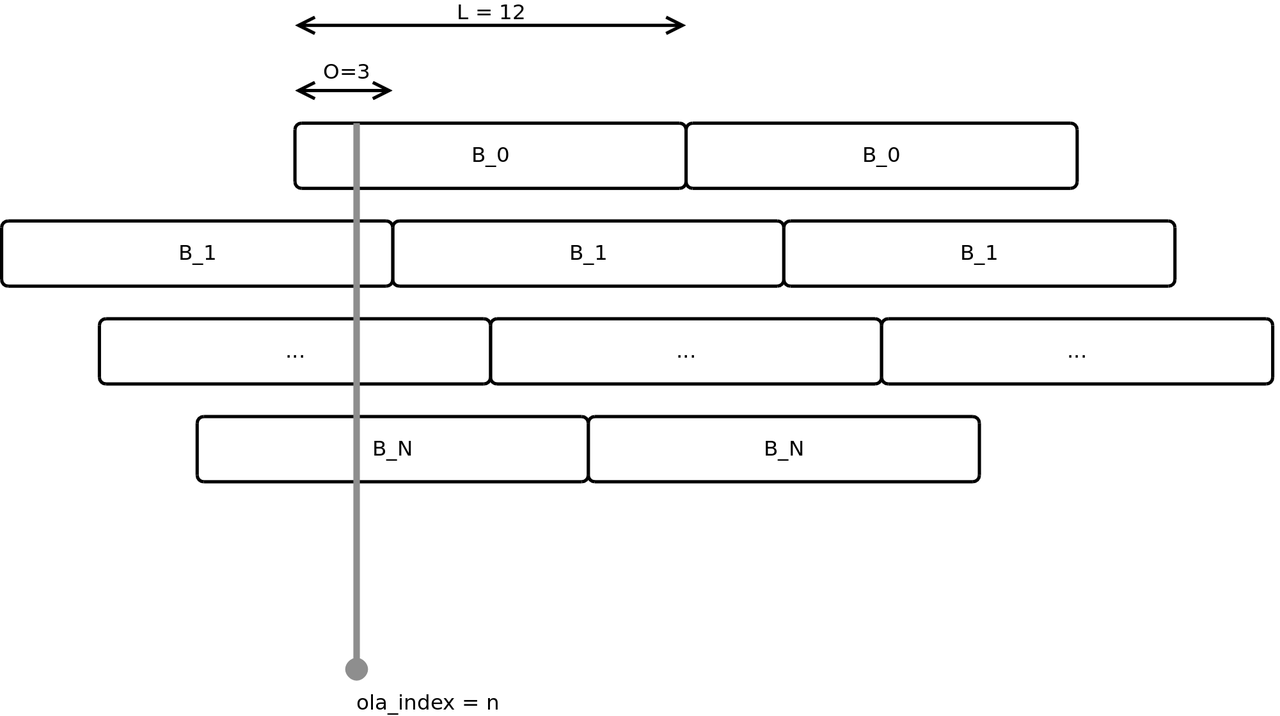# Extensions & Plugins for SuperCollider

## sc3-plugins

The sc3-plugins are a collection of unit generators (UGen), developed by the SC community. For using the full potential of SuperCollider, it is recommended to install them alongside every SC install:

https://github.com/supercollider/sc3-plugins

The sc3-plugins also contain the SC-HOA UGens, which are needed for working with binaural and Ambisonics in SC.

## Extensions

Extra classes are organized in so called Quarks:

https://github.com/supercollider-quarks/quarks

Some addons for SuperCollider require plugins AND extensions. Quarks can be added via code. This example shows how to install the SC-HOA quarks (https://github.com/florian-grond/SC-HOA):

Quarks.install("SC-HOA")

// SuperCollider will automatically install the Quarks here:
Quarks.folder; // execute to see the directory


Another way is to use the Quarks GUI:

Quarks.gui


Warning

The sclang needs to be restarted after installing Quarks and extensions. When doing so, the class library gets recompiled and newly installed classes or unit generators are available.

After restarting sclang (recompiling the class library), you can check whether a Quark (SC-HOA) has been installed properly by checking for related help files, which will be installed:

HOAmbiPanner.help


# Bilinear Transform: RC Lowpass

The bilinear transform is a method for designing digital filters from analog models. More precisely, it brings filters from the Laplace domain to the z-domain. The desired outcome is a difference function, which can be used for the actual filtering process in the discrete time domain.

### Passive RC Lowpass¶

A simple example for the bilinear transform is the RC lowpass:

Transfer Function

The resulting filter has a low pass characteristic with the following frequency response:

In the Laplace domain its transfer function is defined as:

$$H(s) = \frac{\omega_c}{\omega_c + s} = \frac{1}{1 + \frac{s}{\omega_c}}$$

$s$ represents the Laplace operator

$$s = \sigma + j \omega$$

and the cutoff frequency of the passive filter is:

$$\omega_c = \frac{1}{RC}$$

Substitution

For a transform to the z-domain, the Laplace operator $s$ is subsstituted by the $z$ operator:

$$s = \frac{2}{T}\frac{1-z^{-1}}{1+z^{-1}}$$$$z = e^{sT}$$

The following steps rearrange the resulting transfer function to be comprised of summands with the factor $z^{-n}$:

\begin{align} % % H(z) = & \frac{\omega_c}{\omega_c + \frac{2}{T} \frac{1-z^{-1}}{1+z^{-1}}} \\ % = & \frac{\frac{T}{2} \omega_c}{ \frac{T}{2} \omega_c + \frac{1-z^{-1}}{1+z^{-1}}} \\ % = & \frac{\frac{T}{2} \omega_c (1+ z^{-1})}{ \frac{T}{2} \omega_c (1+ z^{-1}) + 1-z^{-1}} \\ % = & \frac{\frac{T}{2} \omega_c + \frac{T}{2} \omega_c z^{-1}}{ \frac{T}{2} \omega_c (1+ z^{-1}) + 1-z^{-1}} \\ % = & \frac{\frac{T}{2} \omega_c + \frac{T}{2} \omega_c z^{-1}}{ \frac{T}{2} \omega_c + \frac{T}{2} \omega_c z^{-1} + 1-z^{-1}} \\ % = & \frac{\frac{T}{2} \omega_c + \frac{T}{2} \omega_c z^{-1}}{1 + \frac{T}{2} \omega_c + (\frac{T}{2} \omega_c -1 )z^{-1}} \\ % \end{align}

For getting the coefficients of our digital filter, the transfer function needs to meet the following strucutre where $a_0=1$:

$$H(z) = \frac{b_0 + b_1 z^{-1}}{1+ a_1 z^{-1}}$$

This is achieved by expanding and simplifying:

\begin{align} % % H(z) = & \frac{\frac{T}{2} \omega_c + \frac{T}{2} \omega_c z^{-1}}{ \underbrace{ \mathbf{\color{gray} 1 + \frac{T}{2} \omega_c }}_{\text{must be 1}} + \left(\frac{T}{2} \omega_c -1 \right)z^{-1}} \\ % = & \frac{\frac{\frac{T}{2} \omega_c}{ 1 + \frac{T}{2} \omega_c} + \frac{ \frac{T}{2} \omega_c}{ 1 + \frac{T}{2} \omega_c} z^{-1}}{1+ \left(\frac{\frac{T}{2} \omega_c}{1 + \frac{T}{2} \omega_c} - \frac{1}{1 + \frac{T}{2} \omega_c} \right)z^{-1}} \\ % = & \frac{\frac{\frac{T}{2} \omega_c}{ 1 + \frac{T}{2} \omega_c} + \frac{ \frac{T}{2} \omega_c}{ 1 + \frac{T}{2} \omega_c} z^{-1}}{1+ \left(\frac{\frac{T}{2} \omega_c -1}{ \frac{T}{2} \omega_c + 1} \right)z^{-1}} \\ \end{align}

Coefficients

Following the above rearrangements, the coefficients can be directly extracted from the transfer funcion:

$$H(z) = \frac{\frac{\frac{T}{2} \omega_c}{ 1 + \frac{T}{2} \omega_c} + \frac{ \frac{T}{2} \omega_c}{ 1 + \frac{T}{2} \omega_c} z^{-1}}{1+ \left(\frac{\frac{T}{2} \omega_c -1}{ \frac{T}{2} \omega_c + 1} \right)z^{-1}} = \frac{b_0 + b_1 z^{-1}}{1+ a_1 z^{-1}}$$\begin{align} \mathbf b_0 = & \frac{\frac{T}{2} \omega_c}{ 1 + \frac{T}{2} \omega_c} \\ \mathbf b_1 = & \frac{\frac{T}{2} \omega_c}{ 1 + \frac{T}{2} \omega_c} \\ \mathbf a_1 = & \frac{\frac{T}{2} \omega_c -1}{ \frac{T}{2} \omega_c + 1} \\ \end{align}

Filter Topology

The original dependence of the electrical components R and C is not important at this point. However, with $\omega_c$ = $2 \pi f_0$ we can get the exact coefficients for any cutoff frequency which can be used for the difference equation or applied in the following topology:

# Configuring the Server

Many application scenarios require specific server configurations. The default server options can be adjusted accordingly.

## Multi IO

The number of audio inputs and outputs can be defined before booting the server. Especially in multichannel applications - like in spatial audio - this is necessary:

s.options.numInputBusChannels  = 16;
s.options.numOutputBusChannels = 48;

// boot with options
s.boot;

// show all IO channels
ServerMeter(s);


# Using Envelopes

The body of a basic electronic kick drum is a sine wave with an exponential decrease in frequency over time. Depending on the taste, this drop happens from about 200-300 Hz to 30-60 Hz. This can be achieved with temporal envelopes.

## Define Envelopes

Before using an envelope, it needs to be defined, using the Env class. It is feature rich and well documented insice the SC help files. The following line creates an exponential envelope with a single segment, defining an exponential drop. It can be plotted using the envelope's plot method:

~env = Env([1, 0.0001], [0.15],\exp);
~env.plot;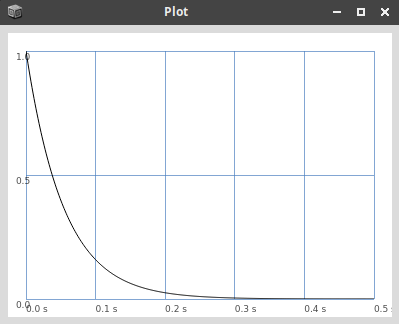---

## Using an Envelope Generator

Envelopes can be passed to envelope generators. The following example generates a control rate signal with the exponential characteristics. It will be sent to the control bus with the index 0 (Out.kr(0,)) and the created node will be freed once the envelope is done, defined by the done action. The bus can be monitored to see the result:

s.scope(1,0,rate:'control')

{Out.kr(0,EnvGen.kr(~env, doneAction: Done.freeSelf))}.play


## A Sine Wave Node

The following node will be used for generating the kick itself. It has two arguments - the gain and the pitch:

(
~synth = {
|gain=0,pitch=100|

// send the signal to the output bus '0'
Out.ar(0, gain*SinOsc.ar(pitch));

}.play;
)


The pitch argument needs to be mapped to the first control bus (0):

~synth.map(\pitch,0);


## Triggering it

The envelope generator line can now be evaluated to retrigger the kick. It will keep on droning, but already has the characteristic punch:

{Out.kr(0,EnvGen.kr(~env, doneAction: Done.freeSelf))}.play


## Exercise

Exercise

Add a second envelope for the gain to stop the kick from droning.

# Icecast

Icecast is a free software solution for creating accessible web-radio streams: https://icecast.org/

It plays nicely with JACK on Linux audio servers, allowing the broadcasting of complex sound synthesis and sonification projects.

# IIR Filters

Infinite Impulse Response (IIR) filters are such filters which include recursion, respectively feedback, in their implementation. Their impulse response does thus not drop to zero after a given time. They can become instable due to the feedback. However, IIR filters require less coefficients and thus less operations to get a filter with the desired effect than FIR filters. As FIR filters, IIR filters create an output sequence $y[n]$ from an input sequence $x[n]$:

### The Difference Equation¶

For the difference equation the recursion is included with additional coefficients $a_n$, feeding back the output signal $y[n]$ with different delays. The following difference function represents a second order IIR filter. Also referred to as biquad filter, this is a basic component for many digital filter implementations:

$$y[n] = b_0 x[n] + b_1 x[n-1]+ b_2 x[n-2] - a_1 y[n-1] - a_2 y[n-2]$$

Or short:

$$y[n] = \sum\limits_{i=0}^{i=N} b_i x[n-i] - \sum\limits_{i=1}^{i=N} a_i y[n-i]$$

### Implementation Structure¶

The above difference equation can be transferred into different implementation structures. These structures can be directly implemented in actual code. The following one is the direct form 1:

### Polynominals¶

A filter of the order $N$ can be expressed as a polynominal, using the Z-operator $z = e^{sT}$:

$$h[n] = \frac{\sum\limits_{n=0}^{n=N} b_n z^{-n}}{\sum\limits_{n=1}^{n=N} a_n z^{-n}}$$

### Pole-Zero Plot¶

IIR filters have roots in the demoninator, called zeros and in the nominator, called poles:

# Additive Synthesis in SuperCollider

## Multichannel Expansion

The following example implements simple additive synthesis in SuperCollider, suited as a starting point for more elaborate designs. Arrays can be helpful for using single UGens multiple times, which is the very idea of additive synthesis. The multichannel expansion allows the use of arrays as arguments for UGens, resulting in an array of objects with the initialization parameters given in the arrays. The following example will create five oscillators, sending their outputs to the buses 0...4:

(
{
|pitch=100|
SinOsc.ar(freq:pitch*[1,2,3,4,5],mul:1/[1,2,3,4,5]);
}.play;
)

s.scope(6,0);


## Mixing

The single partials can be easily summed by wrapping them inside a Mix.ar() UGen. They are now all sent to the first output bus:

(
{
|pitch=100|
Mix.ar(SinOsc.ar(freq:pitch*[1,2,3,4,5],mul:1/[1,2,3,4,5]));
}.play;
)

s.scope(6,0);


## Dynamic Allocation

For more partials and dynamic sizes, it makes sense to use the fill() method of the array class:

(

~n_part    = 30;

|pitch=100|

Mix.ar(
SinOsc.ar(
freq:pitch*Array.fill(~n_part,{arg i; (i+1)}),
mul: Array.fill(~n_part,{arg i; 1/(((i)+1))})
)
);

}.play;
)


## Exercises

Exercise I

Adjust the partial weight formula to generate basic waveforms (square, sawtooth, triangle). Monitor the signal in the scope to verify. Try different numbers of partials and compare the results.

Exercise II

Combine the example with a mouse control, allowing to change two parameters of the timbre or partial frequencies.

# Patches and Subpatches in Pure Data

## Arguments

The following examples are based on patches and additional files, called abstractions. To make them work, all involved patches need to be located in the same direction (by cloning the complete repository). Arguments are passed to objects after the name, separated by a white space. The patch arguments-help.pd shows this by creating an arguments object: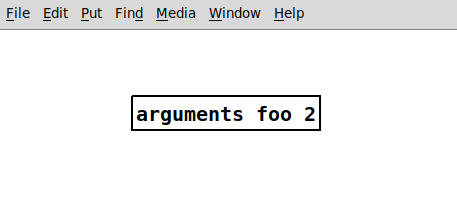Inside an abstraction, individual arguments can be accessed with the $ operator and their index. The loadbang is executed on the object's creation, thus printing both arguments on start. This is helpful for setting initial values in patches, as shown in arguments-help. Once created, it will print the arguments to the main Pd window: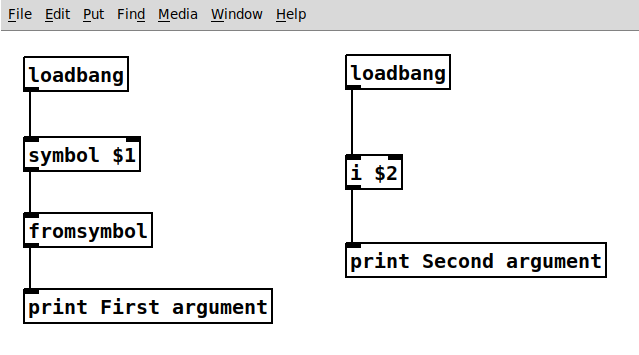## Subpatches Subpatches can be very helpful for creating cleaner patches without addtional abstractions and files. To create a subpatch, use the object pd with an optional string argument for naming the subpatch. They can be used like abstractions but do not require an additional file. ### Graph-on-Parent When toggling Graph-on-Parent in an object's properties, it can expose GUI elements to its parent patch. This is a good way of cleaning your patch and showing only what is needed in a performance situation. It works for both abstractions and subpatches. The example patches.pd makes use of this to create a filter subpatch with controls. The left hand audio input of the suppatch is a fixed frequency sawtooth. The right hand control input sets the Q of the filter.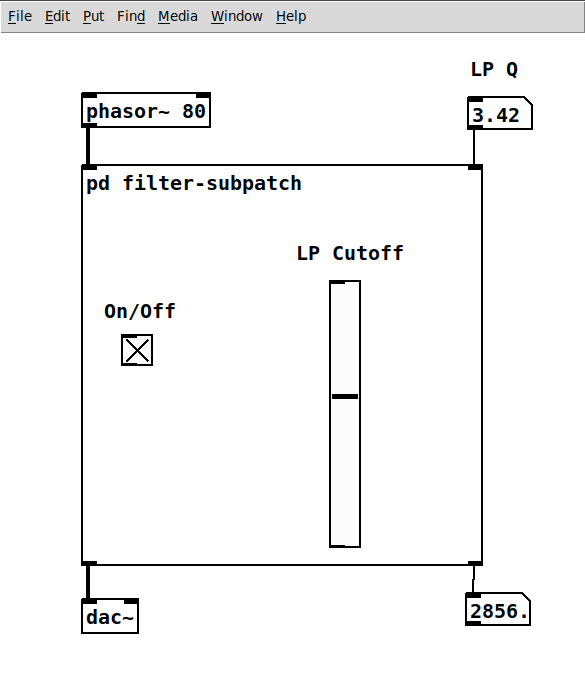On the inside, the moog~ object is used. It is not part of PD vanilla and can be installed with the flatspace ggee extensions from Deken. The red rectangle marks the area visual in the parent patch. All GUI components inside this area will be visible: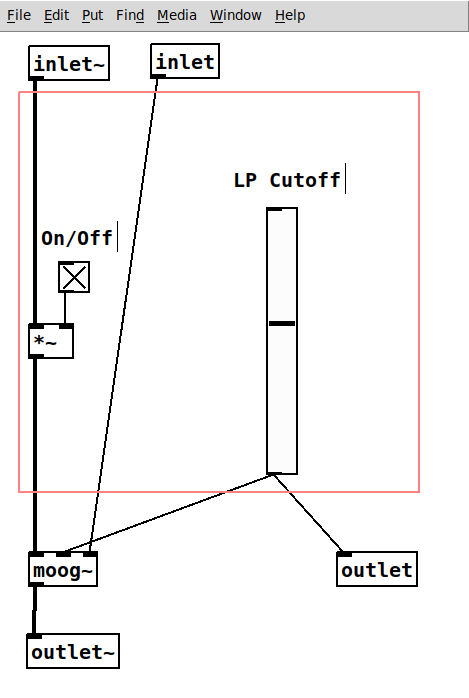## Inlets and Outlets The patch has two inlets - one in audio rate (inlet~) and one in control rate - and two outlets, also with audio rate (outlet~) and control rate. For inlets and outlets, their horizontal order determines their order in the object when patched from the parent. Changing them can mess up the complete patching. # FIR Filters Finite Impulse Response (FIR) filters are such digital filters which have - as the name suggests - a finite impulse response. These filters do not have an internal feedback and can be applied simply by convolving an input sequence$x[n]$with the filter's impulse response$h[n]$to get the output sequence$y[n]$: For FIR filters, the impulse response$h[n]$is a one-dimensional array containing the$N$filter coefficients. The following example shows the coefficients, respectively the impulse response for a filter of the order$N=5$: ### The Difference Equation¶ From the impulse response we can get the coefficients$b_n$to write the difference equation, which is the basis for applying a filter: $$y[n] = b_0 x[n] + b_1 x[n-1]+ b_2 x[n-2]+ b_3 x[n-3]+ b_4 x[n-4] + b_5 x[n-5]$$ ### Implementation Structure¶ Another important representation of a filter is the implementation structure. It can be directly derived from the difference equation. Each$z^{-1}$block represents a delay by one sample. This case shows the direct form: ### Applying a Filter by Convolution¶ Applying the filter with the given impulse response is done by simple convolution, which is the same as passing samples from the input of the implemtation structure above to its output. The above equation is then written as follows: $$\begin{eqnarray} y[m] &=& x[n] * h[m]\\ &=& \sum\limits_{m=0}^{M} h[m] x(n-m)\\ &=& \sum\limits_{m=0}^{M} b_m x(n-m)\\ \end{eqnarray}$$ ### The Transfer Function¶ The difference equation can also be expressed as a sum: $$h[n] = \sum\limits_{n=0}^{N} b_n x[n-1]$$ The transfer function of the filter can be obtained via the z-transform: $$H[z] = \sum\limits_{n=0}^{N} h[n] z^{-n}$$$$H(z) = 0.5 z^{-2} + z^{-3} + 0.5 z^{-4}$$ In the next step, all exponents are shifted by expanding with$z^{4}$: $$H(z) = \frac{z^2 + 2z + 1}{z^4}$$ Factor the denominator to find its roots: $$H(z) = \frac{(z+1)(z+1)}{z^4}$$ There is a double zero at$\mathbf{z=-1}$. As all causal FIR filters it has$N=4$poles at the origin$z=0$. With$z=e^{j\omega}\$ the complex roots can be obtained:

$$Z = (e^{-i\omega} +1)(e^{-i\omega} +1)$$

## In the Unit Cirlce¶

An LTI system can be visualized in the z-plane by plotting the zeros, respectively the roots of its impulse response.

### Frequency Response¶

The frequency response of the filter

# FFT-IFFT & Overlap-Add